Next: Pseudo-random number generators Up: Probabilistic Encryption Previous: Semantic Security   Contents

Subsections

## How to play poker over the telephone

We will not analyze an entire game of poker, but just the task of each player [we will assume only two players] getting dealt cards so that (i) each player gets his cards at random, with all cards equally likely (ii) neither player knows what his opponent has (iii) the players cannot get the same cards. You will probably appreciate the procedure more if you first try to devise a way of doing this yourself.

Several previous attempts to use cryptographic devices for this purpose were flawed12. The elaborate procedure we describe is based on some number-theory tools developed in section 3.3 and earlier in this section:

1. Ifand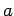is a square mod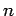, it has four square roots. If we know roots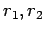with, we can find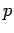,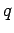.
2. If,is a square modif and only if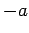is not a square (Lemma 22). If we also have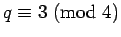, then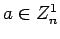if and only if.
3. We can test whether or notwithout knowing.

Two techniques are used repeatedly. They are also of interest in other applications.

Theorem 27 (random numbers)   B can generate a random number so that A does not know its value now, but can verify it later.

A first try'' might be for B to generate a random number and give an encryption of it to A, with the key revealed for verification later. This does not work, since A cannot be sure that B chose his number at random.

To insure randomness, A gives B a second number (which A is supposed to choose at random) after receiving B's encryption, and the number used by B is the exclusive or'' of the two:

 A chooses 0110001 B chooses 1011011 B uses 1101010
Even if one of the players does not choose his number at random, the result will be random as long as the other player does.

Theorem 28   B can ask A a question related to. The answer to this question may or may not allow B to factor. At the time the question is asked, A cannot tell whether the answer he gives B is useful or useless, but this can be verified later.

Proof: A chooses primes, and announces. Using the technique of Theorem 27, B generates a random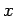, and will ask A for a square root of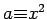. At the time the question is asked, A will knowbut not. B is allowed to specify whether the square root A gives him is or is not in.

Ifand B specifies that the square root is in, A will give B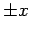, which is useless. B can get useful information by specifying that the square root is not in. If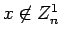, the square root inwill be useful, and the other will be useless.

Sinceis randomly chosen, and half the possibleare inand half are not, A will not be able to guess right more than half the time whether he is being asked for useful or useless information.

### The procedure

1. A announces, each of which is a product of two large primes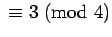. He encodes the names of the different cards using different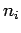and also announces these. [if B finds the factors of one of the, it does not help him identify the other cards] B does the same thing using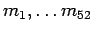.
2. To get a card, B asks A one question for each, using the procedure of Theorem 28. 51 of the questions will be useless. The useful question allows B to decode the name of the card he receives. [it is crucial that A will be able to verify the uselessness of the other 51 questions after the game.]
3. B deletes the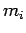corresponding to the card he received (this ensures A will not get this card).
4. A gets a card by asking 51 questions about the remaining, of which 50 are useless. He deletes thecorresponding to this card.
5. If B gets a second card, he asks 51 questions. He avoids getting the same card twice by not asking a useful question about the sameas the first time.
This procedure is too cumbersome to be practical, but it is a good example of the kinds of things that can be done using cryptographic procedures. Current research focusses on other tasks involving exchanges of encrypted and partially encrypted information between two players.

#### Footnotes

... flawed12
R. Lipton, How to cheat at mental poker,'' Proceedings of AMS Short Course on CryptographyNext: Pseudo-random number generators Up: Probabilistic Encryption Previous: Semantic Security   Contents
Translated from LaTeX by Scott Sutherland
2002-12-14